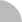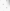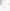##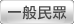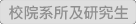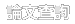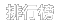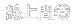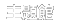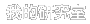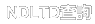(3.238.96.184) 您好！臺灣時間：2021/05/18 15:26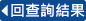### 詳目顯示:::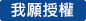: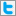Twitter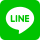•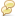被引用:0
•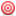點閱:103
•評分: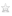•下載:0
•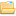書目收藏:0
 本論文提出，用多項式加速法來加速基本迭代法，以解大型線性系統 Au= b，其中 A 為 n 階 的對稱正定矩陣， b 是給定的 n 維實向量。出發點 是找 一個實係數 m 次多項式 P_m(x) 且 P_m(1)=1，使得 .epsilon.^( m) = P_m(G).epsilon.^(0)，其中 .epsilon.^(m)為第 m次迭代誤差向量 ，而G為基本迭代法中的迭代矩陣。如此的迭代演算方式，僅涉及矩陣乘 向量和向量的相加，所以，非常適合用向量電腦及平行電腦處理。本文用 Dirichlet核、F'ejer核和 Legendre多項式來產生P_m(x)，以建構出各別 的多項式加速法，並與現有的Chebyshev 多項式加速法做比較。並利用 VAX 4500及IBM ES 9000/860 做數值實驗，其中後者為向量電腦。如此， 可以比較，各多項式加速法在一般序列式電腦及向量式電腦處理之優劣性 。
 In this paper, we studied the way of using different polynomials to accelerate basic iterative methods for solving large linear system Au=b, where A is n by n spd matrix, and b is a given n dimension real vector. The viewpoint is to find a polynomial of degree m, with P_m(1)=1, such that .epsilon.^(m)= P_m(G).epsilon.^(0), where .epsilon.^(m) is the mth error vector. Here, the matrix G is the iteration matrix. Such algorithms involved only matrix vector multiplications, and vectors additions, so they are well suited for vector and parallel computers. Polynomials used are generated from the F' ejer kernel, Dirichlet kernel and Legendre polynomial and we also compared them with the Chebyshev acceleration.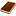國圖紙本論文推文當script無法執行時可按︰推文 網路書籤當script無法執行時可按︰網路書籤 推薦當script無法執行時可按︰推薦 評分當script無法執行時可按︰評分 引用網址當script無法執行時可按︰引用網址 轉寄當script無法執行時可按︰轉寄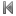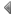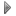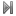top
 無相關論文

 無相關期刊

 1 透過平衡態生化反應之多項式取值 2 圖的特徵多項式之探討 3 水泥質材料加速氯離子傳輸試驗之加速時間與鹽水浸漬試驗浸漬時間之關係 4 國中生在多項式乘除運算的主要錯誤類型及其補救教學之研究 5 一個攻擊樹之延伸研究 6 「同儕個別學習輔導」融入「多項式運算」補救教學之研究 7 加速碳酸化對轉爐石中含鈣物種轉化反應之影響 8 資訊融入數學合作學習對國中二年級多項式與其加減及多項式乘除單元學習成效之影響 9 高一學生學習多項式不等式單元錯誤類型分析 10 高雄地區高一學生多項式函數與圖形單元錯誤類型分析 11 透過高頻電磁波加速電子來間接加速質子的數值模擬研究 12 虛擬教具應用於國中學生學習多項式展開與因式分解之影響 13 擬固定多項式問題研究 14 二項式理想在多項式環和Laurent多項式環的討論 15 RFIDEPCglobal系統與UbiquitousID系統之整合機制研究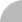簡易查詢 | 進階查詢 | 熱門排行 | 我的研究室